Easy to understandbuttricky to calculate

The IRR measure is an annual percentage return that is calculated by comparing the benefits from a spend decision against the costs and expressing this result as an annual compounded % rate. Most people can see something similar everyday - the annual rate of return on a loan, or a quoted interest rate on a credit card - but in reality the calculation of IRR is not at all straightforward.

A seasoned CFO will need to know the IRR answer and will be able to quickly identify the correct IRR calculation so it is important to calculate it correctly.

In this white paper we examine two of the common pitfalls with IRR and discuss the technique used in Shark which avoids these errors.What is IRR?

Internal Rate of Return when used for business case decisions is a measure of the annual % rate of profitability on a project or solution when compared to the original amount spent or invested. It is particularly useful in comparing the relative merits of different projects which all have different IRR values. As a rule, the higher the IRR % the better a project is for the investor. In addition, an investor can compare the project IRR generated against their own internally required minimum return rate (sometimes known as the hurdle rate) to check whether a project should be approved.

More formally IRR is defined as the return rate per annum taking into account the initial and recurring costs of the solution and comparing these to the timing of benefits identified as a result of implementing the project.

With an internationally recognised method of calculation defined by the finance community you can rely on IRR to be solution independent. This means that in a sales environment all you have to worry about is whether the size and timing of the costs and the benefits have been described correctly.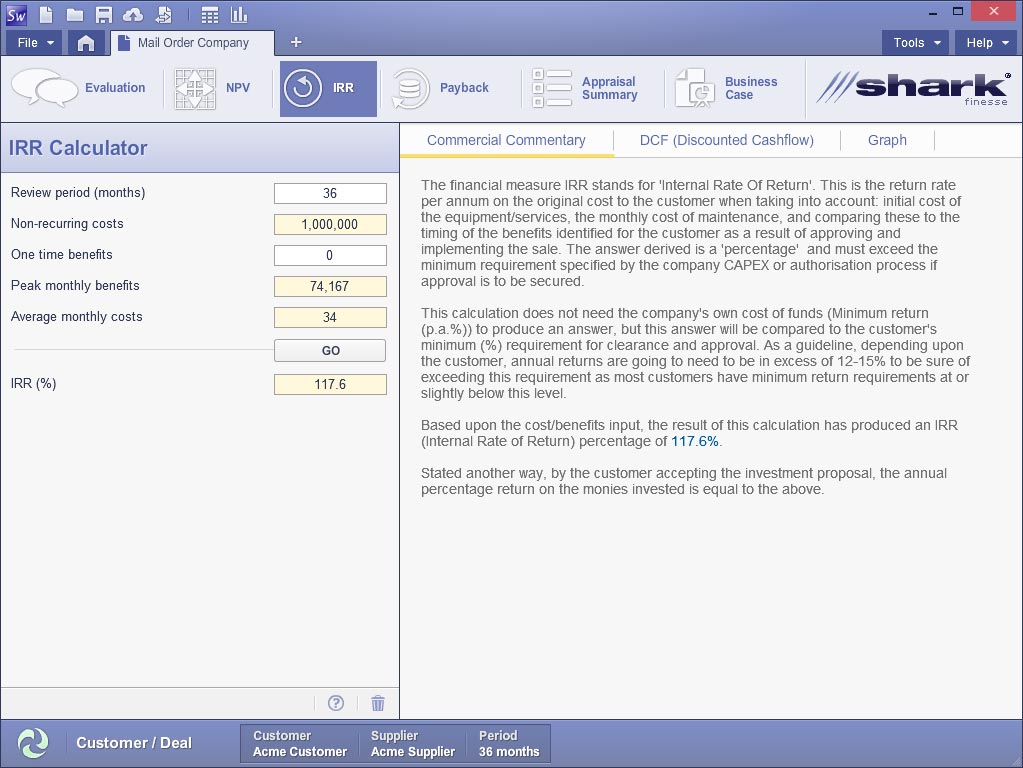IRRa member of the ROI family

IRR is part of the family of ROI measures which also includes Net Present Value (the future profit expressed in today’s terms) and Payback (the time taken to repay the initial investment). One quick way of checking that the calculated IRR is correct for a project is to insert the IRR value answer into the calculation for the NPV. The NPV when calculated with the IRR as the minimum rate of return or discount rate % should be close to depending on how many decimal places are used in the calculation).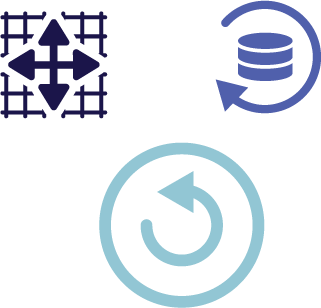Pitfall 1 the simple percentage "return" comparison

A question that sometimes arises is, "why not just use a simple percentage that can be created by dividing the total value of the benefits by the total costs"? There is a common misconception that a simple percentage return should be just as useful as IRR. However, IRR is more sophisticated than this simple calculation as it describes the percentage return on an annual basis and this makes it ideal for comparing investments in different projects. The simple percentage approach is inadequate because it does not include this information about the time period over which the benefits are received and the costs are incurred. However, this does mean that the timing of the benefits and costs is critical for a meaningful IRR that the investor can use. Take a look at the following examples:

Example 1

Spend \$100 today Receive \$120 in twelve months

The simple percentage calculation here ((120-100)/100) gives the rate of return over a year of 20%. This is no surprise that it’s the same result as the IRR calculation because the base period is one year!

Example 2

Spend \$100 today Receive \$120 in six months

The simple percentage calculation ((120-100)/100) gives the rate of return over a year of 20% which is the same as example 1. However, the IRR calculation would produce a value of 44% per annum which is more than double the simple calculation. Halving the time to get the benefits should reasonably be expected to double the answer but because IRR is a compounded rate it is actually even greater. 20% yield is more quickly achieved within the first six months but because IRR is always expressed as an annual percentage equivalent return then the calculation must convert this into a 12 month valuation / expression. As such, expectation for an annual valuation is that another 20% will be achieved in the second six months. Not only that, but there will be an extra 20% of the first 20% i.e. 4% making the total 44%.

As we can see, the IRR depends heavily on the timing of the receipt of the \$120 gross benefit. This actually gives us an advantage when using Shark to produce an IRR because all benefits (and costs) are individually profiled and valued on a monthly basis making the IRR very accurate. The added benefit of using Shark to profile the benefits is that customer sponsorship is easier if the start date for a benefit can be adjusted to suit the real world onset of that benefit For example it would be impossible to receive benefits in the first month from a system that takes 6 months to implement. It is important to understand that for a business case to be credible it must contain realistic, credible and sponsored timing of costs and benefits. Customers are reluctant to sponsor a ‘general year 1 saving’!

Pitfall 2 using Excel to calculate IRR

One step further from the simple percentage return described above is to use common spread sheet-style programs to calculate IRR, e.g. Microsoft ©Excel.

Example 3

A simple IRR in Excel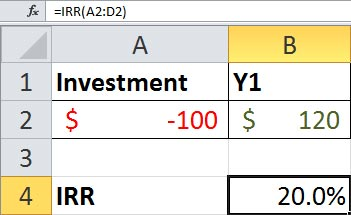Example 3 is exactly the same as example 1 translated into Excel and it gives the correct answer of 20%. However, we can see immediately that it is impossible to represent example 2 in Excel because there is no way to put the \$120 benefit in anything other than year 1 (Y1). Excel has assumed that all the \$120 occurs on day 365 of each year in a single lump sum – not only is this inelegant but it is plainly wrong in the real life!

The Excel IRR result is only correct for the specific case where cashflows occur once at the end of the year. If we compare example 2 (44%) with example 3 (20%) another potentially more important factor may come into play. If the business case depends on the IRR for approval, using Excel could result in rejection simply because the result is too low compared to the expected value.

The solution using Shark to calculate correct IRR

In Shark every single benefit Evaluator (same for the costs) creates its own individual monthly cash flow. As we have highlighted above, this timing information is important when gaining customer sponsorship because a delayed benefit is easier to support than one which is claimed to start immediately.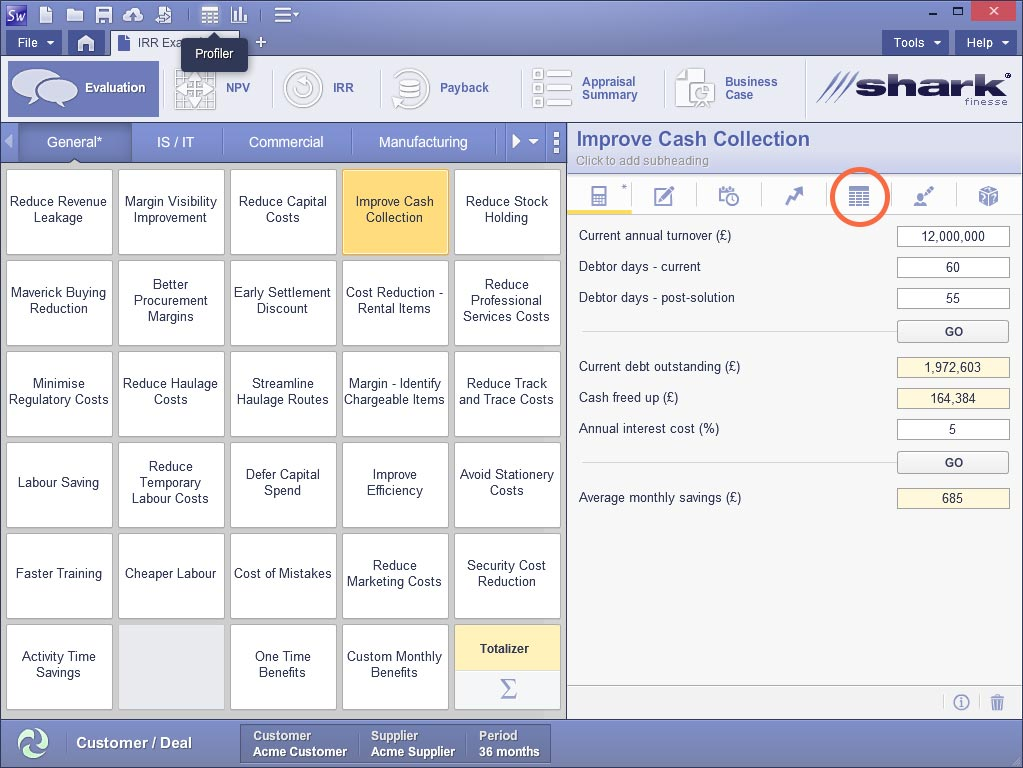You can see an individual Evaluator cashflow by clicking on the icon highlighted.

Note that the average monthly savings are 685 x 30/36 = \$571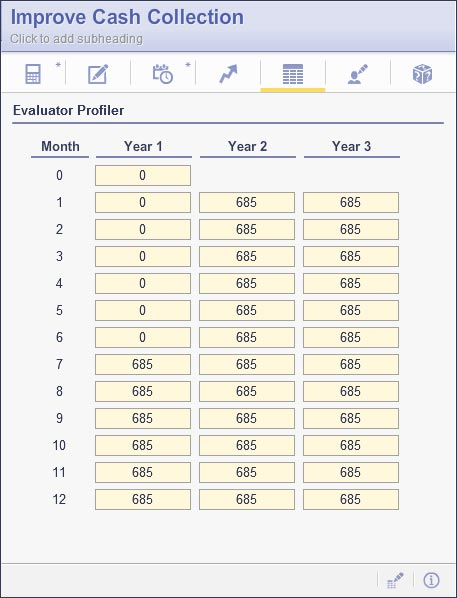Shark also provides a full net cash flow which combines all costs and benefits. You can click on the similar icon at the top of the evaluator page and/or produce a report with the NPV cash flow providing you set the minimum return rate (discount rate in the customer deal window) to zero.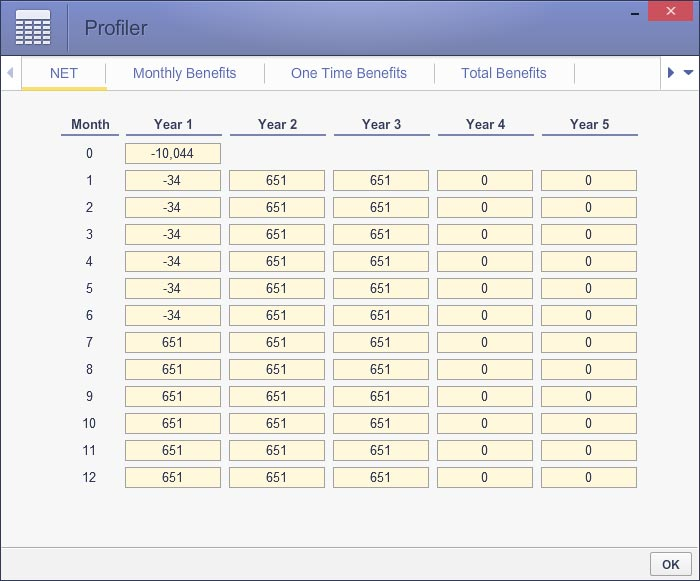Trying to put Shark values back into Excel to give a similiar IRR

It is sometimes tempting to try and recreate the IRR from Shark in Excel but because of the lack of timing detail this is not possible. Rather than trying to compress Shark to conform to Excel by working out annual values, the above monthly cash flow really needs to be operated on to give the true IRR. But as we have seen Excel does not have the true monthly IRR function so this is not really an option.

Putting the numbers back from Excel into Shark

Shark is much more capable than Excel so it is easier to work back from Excel if you have compressed the numbers into yearly values. In this instance, the IRR produced by Shark will match the Excel but the IRR result will inevitably be wrong because the monthly timing information is not included.

Conclusion

IRR is not an easy concept to follow sometimes. It can lead to very high percentages where the benefits arise very quickly and there are very low upfront costs. Depending on how you manage the timing of the costs and benefits you can arrive at misleading results. The only way to ensure client sponsorship for the business case is to value costs and benefits in the months they occur – this is handled transparently and seamlessly in Shark.

Contact Shark Finesse

Contact Info

Shark Finesse Ltd.
Grove House
Lutyens Close
Basingstoke
Hampshire
RG24 8AG
United Kingdom
• +44 (0) 1256 338635
• sales@sharkfinesse.com